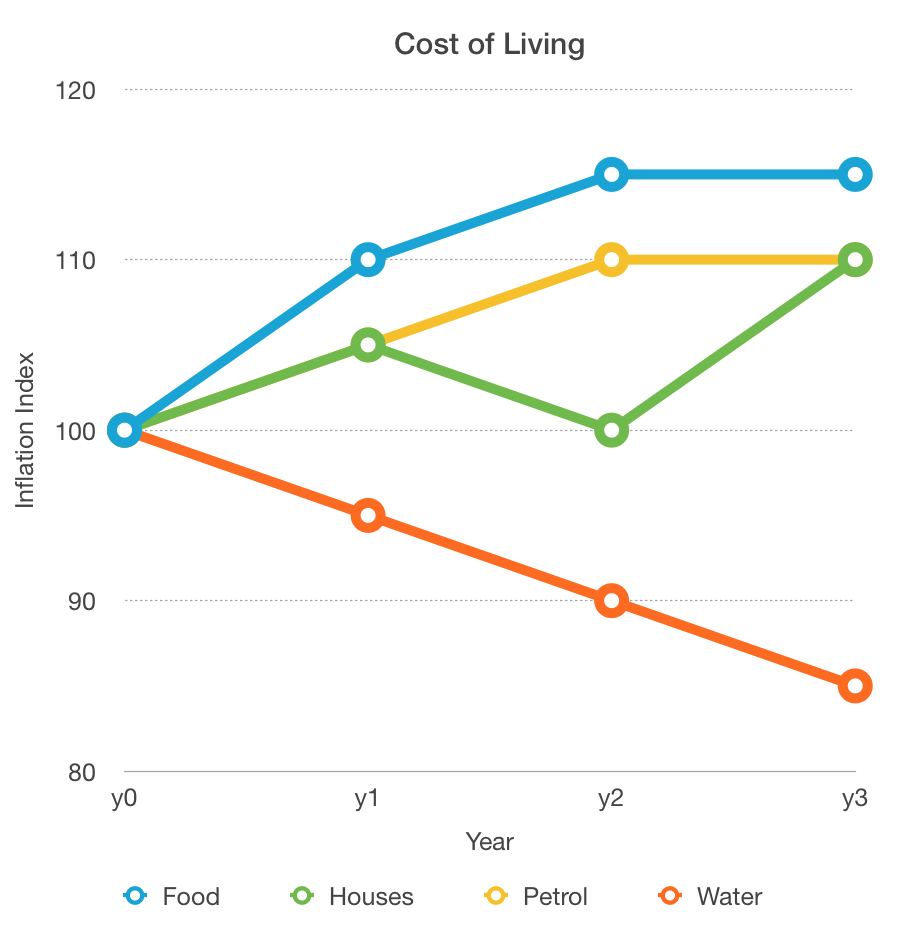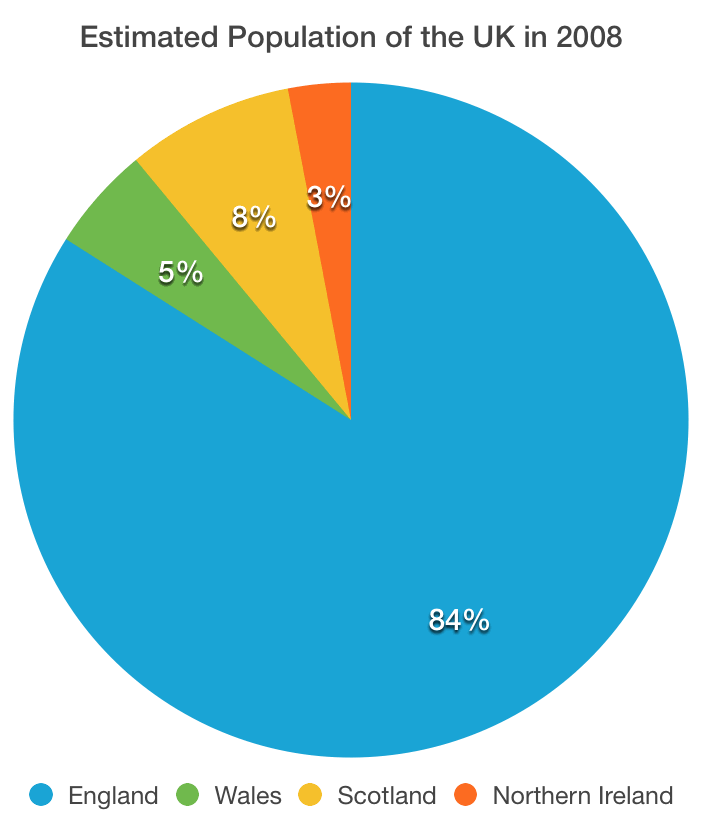Numerical Reasoning Practice Test

This is a practice numerical reasoning test that simulates a real numerical reasoning aptitude test.

• The test has 10 questions and you should aim to complete the test within 10 minutes.
• All questions are multiple-choice.
• Answers and explanations to the questions are provided at the end of the test.
Powered byNumerical Reasoning: question 1 of 10Which period saw the greatest percentage decrease in water prices?

Numerical Reasoning: question 2 of 10If a house cost £300,000 at the end of y1, what will it cost at the end of y3?

Numerical Reasoning: question 3 of 10If the price of food was rebased in y1, what would the inflation index be for y3?

Numerical Reasoning: question 4 of 10Which product experienced the biggest change in percentage terms in y1-y2?

Numerical Reasoning: question 5 of 10The population of the UK in 2008 was 60,587,300. What was the population of Northern Ireland, to the nearest thousand?

Numerical Reasoning: question 6 of 10The population of the UK in 2008 was 60,587,300. If the population of the UK without England was considered, what percentage would Wales make up?

Numerical Reasoning: question 7 of 10The population of the UK in 2008 was 60,587,300. If the population of the UK grew on average 6% during 2008, what would you estimate the population of Scotland to be in 2009?

Numerical Reasoning: question 8 of 10The population of the UK in 2008 was 60,587,300. If the population of Scotland was evenly distributed to the remaining 3 countries, what would be the population of Northern Ireland?

Numerical Reasoning: question 9 of 10The population of the UK in 2008 was 60,587,300. If a new country joined the UK, of population 13 million, what percentage of the UK population would England make up?

Numerical Reasoning: question 10 of 10The population of the UK in 2008 was 60,587,300. What is the ratio of people living in England to those living in Scotland?